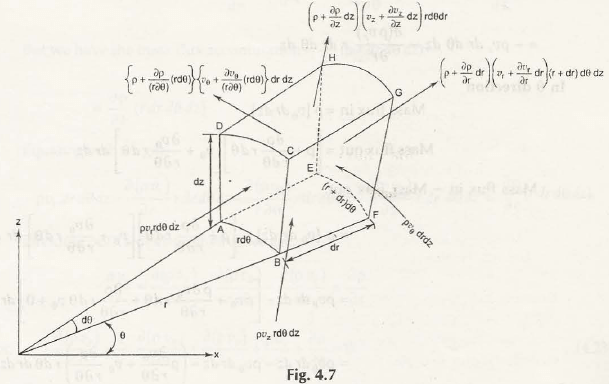# Continuity Equation in a Cylindrical Polar Coordinate System

We have already derived the continuity equation in three dimensions in differential form. It is also possible to get continuity equation using the same system for all flows in a cylindrical polar coordinate system as shown in Fig. 4.7Suppose the coordinate system of an elementary control volume be (r, θ, z)

In the direction of r, the Rate of mass enters the system can be given by ρvr rdθdz

Rate of mass leaves the system be (ρ + ∂ρ/∂r  dr)(v_r  + (∂v_r)/∂r  dr) rdθdz

Since,

For control volume:

Rate of mass entering control volume = Rate of mass leaves + Rate of accumulation of mass

Substituting the values, we get-

ρvrrdθdz    =    (ρ + ∂ρ/∂r  dr )(v_r  + (∂v_r)/∂r  dr )rdθdz    +    Rate of accumulation of mass

Or,

Rate of accumulation

of mass    =    ρ [vrrdθdz] – (ρ + ∂ρ/∂r  dr )(v_r  + (∂v_r)/∂r  dr )rdθdz

=    ρ [vrrdθdz] – [ρvr + ρ (∂v_r)/∂r  dr + vr∂ρ/∂r  dr + ∂ρ/∂r  .  (∂v_r)/∂r(dr)2] [rdθdz + drdθdz]

Neglecting higher order terms, so ∂ρ/∂r  .  (∂v_r)/∂r(dr)2 = 0

Or,
=    ρ [vr rdθdz] – [ρvr + ρ (∂v_r)/∂r  dr + vr∂ρ/∂r  dr] [rdθdz + dr dθdz]

=    ρ [vr rdθdz] –[ρvrrdθdz+ ρ (∂v_r)/∂r rdrdθ dz + vr∂ρ/∂r rdrdθ dz

+ ρvrdrdθdz]

(Other higher order terms are neglected)

=    ρvrdr dθdz – (ρ (∂v_r)/∂r  + v_r  ∂ρ/∂r)rdrdθdz

Or,

Rate of accumulation of mass    =    – ρvrdr dθdz– ρ (∂v_r)/∂rrdr dθdz

Now,

Rate of accumulation of mass in θ direction can be given by,

Rate of mass enters beρv_θdrdz

Rate of mass leaves be (ρ + ∂ρ/r∂θ  dθ)(v_θ  + (∂v_θ)/r∂θ  dθ)drdz

So,

Rate of accumulation of mass = ρv_θdrdz– (ρ + ∂ρ/r∂θ  dθ)(v_θ  + (∂v_θ)/r∂θ  dθ)drdz

= ρv_θdrdz–[ρv_θ+ρ (∂v_θ)/r∂θ+v_θ  ∂ρ/r∂θ  dθ+0] dr dz

(Neglecting higher order terms, so we can write zero ‘0’)

= ρv_θdrdz–ρv_θdrdz + (ρ (∂v_θ)/r∂θ+v_θ  ∂ρ/r∂θ  dθ)drdz

Or,

Rate of accumulation of mass =– (∂(ρv_θ))/r∂θrdr dθdz

Similarly,

Rate of accumulation of mass in z direction can be given by,

Rate of mass enters be ρv_zrdθdr

Rate of mass leaves be (ρ + ∂ρ/r∂z  dz)(v_z  + (∂v_z)/∂z  dz)rdθdr

So,

Rate of accumulation of mass = ρv_zrdθdr– (ρ + ∂ρ/r∂z  dz )(v_z  + (∂v_z)/∂z  dz)r dθdr

= ρv_zrdθdr–[ρv_z+ v_z  ∂ρ/r∂z  dz+ρ (∂v_z)/∂z  dz+0]r dθdr

(Neglecting higher order terms, so we can write zero ‘0’)

= ρv_zrdθdr– ρv_zrdθdr –(ρ (∂v_z)/∂z+v_z  ∂ρ/∂z)r dθdrdz

Or,

Rate of accumulation of mass = –(∂(ρv_z))/∂zrdr dθdz

Adding all above equations of rate of accumulation of mass, we get the rate of accumulation of an elementary control volume –

= –ρvrdr dθdz– ρ (∂v_r)/∂rrdr dθdz– (∂(ρv_θ))/r∂θrdr dθdz– (∂(ρv_z))/∂zrdr dθdz

Or,

∂ρ/∂trdr dθdz = – [(ρv_r)/r  – ρ (∂v_r)/∂r– (∂(ρv_θ))/r∂θ– (∂(ρv_z))/∂z]rdr dθdz

Or,

∂ρ/∂t = – [(ρv_r)/r  – ρ (∂v_r)/∂r– (∂(ρv_θ))/r∂θ– (∂(ρv_z))/∂z]

= –[(∂(〖ρv〗_r))/∂r  – 1/r.(∂(ρv_θ))/∂θ–(∂(ρv_z))/∂z  – (ρv_r)/r]
Or,

∂ρ/∂t + [(∂(〖ρv〗_r))/∂r+1/r.(∂(ρv_θ))/∂θ+(∂(ρv_z))/∂z+(ρv_r)/r] = 0

This is the three-dimensional Continuity Equation for unsteady compressible flow.

Note:
The above Continuity Equation can be formed for two-dimensionalcoordinate system (r, θ) for different fluid flow-

For Unsteady Compressible Flow:

The equation can be written as-

∂ρ/∂t + [(∂(〖ρv〗_r))/∂r+ 1/r.(∂(ρv_θ))/∂θ+ (ρv_r)/r] = 0

For Steady Compressible Flow:

The equation can be written as,

(∂(〖ρv〗_r))/∂r+ 1/r.(∂(ρv_θ))/∂θ+ (ρv_r)/r=  0

For Unsteady Incompressible Flow:

The equation can be given by,

(∂(v_r))/∂r+ 1/r.(∂(v_θ))/∂θ+ v_r/r=  0

For Steady Incompressible Flow:

Equation should be-

(∂(v_r))/∂r+ 1/r.(∂(v_θ))/∂θ+ v_r/r=  0

Links of Previous Main Topic:-

Links of Next Mechanical Engineering Topics:-

Trustpilot

We’re rated Excellent

4.7 out of 5 based on 39 reviews

## Join over 200,000+ happy students served our tutors

Take a look at independent reviews to see what users have to say about our homework help service.## 4.5/5

overall rating
on LiveChat## 96%

of users
recommend service

## How It Works

1. Get Price Estimate.
1. Get Price Estimate.#### Submit.

Send us your homework. Mention deadline & upload relevant files.

2. Pay Securely.
2. Pay Securely.#### Pay.

We accept all major credit cards. Partial payments also accepted.

3. Get Solutions.
3. Get Solutions.#### Delivered

Receive error-free, step-by-step solutions in your email.

## Why use Myhomeworkhelp?

We provide you with the homework help from top experienced experts. Your satisfaction is a priority to us. Get better grades or money-back. It’s that risk-free! Furthermore, everything about you is kept confidential.

## How quickly can you help me with my homework?

We take pride in our 24×7 homework help services. Which means, student can approach us anytime, to get help even on short notices. As short as few hours! And yes, we provide complementary plagiarism-free report.

## How much will it cost?

We do not have a monthly fees or minimum payments. Each homework task is quoted a unique price. It is based on tutor’s estimate of the time it will take them to complete your task. There are no obligations, and you are free to discuss the price quote with the tutor.
We do accept partial payment (subject to our policy) to start working on your assignment help. You can pay the remaining amount when your task gets completed. No pressure of up-front payment. No hidden costs.

## Who are your tutors?

All our experts are highly qualified professionals – holding at least Master’s degree of their respective fields of expertise. We are very selective and choose only the best qualified tutors for each academic subject. All tutor applicants must provide academic transcripts for each degree they hold, and are tested and screened carefully by our staff.

## I have privacy concerns. Can I trust you?

Myhomeworkhelp has completed over 250,000 homework help tasks over the last 12 years. But do not take our word for it! See our here and on Trustpilot. Furthermore, everything about you is kept confidential. Your credit card information is not stored anywhere, and use of PayPal relies on their secure payment networks. Your identity, payment and homework help are in safe hands.

#### Check our video

##### Our Reviews